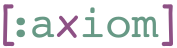## 1    build-plan: Sorts Files by Dependencies

For the examples in this section we create a few dummy source files using this function:

``````(defn create-example-file [name content]
(with-open [file (io/writer name)]
(doseq [expr content]
(.write file (pr-str expr)))
(io/file name)))

(def example-dir "/tmp/permacode.publish/example")
(.mkdirs (io/file example-dir))
(def foo (create-example-file (str example-dir "/foo.clj")
'[(ns example.foo
(:require [permacode.core :as perm]))
(perm/pure
(some-thing))]))

(def bar (create-example-file (str example-dir "/bar.clj")
'[(ns example.bar
(:require [permacode.core :as perm])
(:require [example.foo]))
(perm/pure
(some-thing-else))]))

(def baz (create-example-file (str example-dir "/baz.clj")
'[(ns example.baz
(:require [permacode.core :as perm])
(:require [example.bar]))
(perm/pure
(some-thing-else2))]))``````

Given these files, `build-plan` should return these files in order of their dependencies.

``(build-plan [baz foo bar]) => [foo bar baz]``

In case of an unmet dependency, a proper error message is presented.

``(build-plan [bar baz]) => (throws "Unmet dependency: example.foo")``

Supported namespaces (`permacode.validate/white-listed-ns`) are ignored.

``````(def foo2 (create-example-file (str example-dir "/foo2.clj")
'[(ns example.foo2
(:require [permacode.core :as perm])
(:require [clojure.string :as str]))
(perm/pure
(some-thing))]))``````
``(build-plan [foo2]) => [foo2]``

Additionally, permacode hashes are also ignored.

``````(def foo3 (create-example-file (str example-dir "/foo3.clj")
'[(ns example.foo3
(:require [permacode.core :as perm])
(:require [perm.FOOBARBAZQUUX]))
(perm/pure
(some-thing))]))``````
``(build-plan [foo3]) => [foo3]``

### 1.1    Under the Hood

The helper function `get-ns` takes a file object and returns its first expression. For example:

``````(get-ns bar) => '(ns example.bar
(:require [permacode.core :as perm])
(:require [example.foo]))``````

## 2    hash-file: Hash a Source File

Now that we have our sorted list of files we can go one-by-one and hash it.

``(def hasher (hasher/nippy-multi-hasher (hasher/atom-store)))``

For this, we start with a map containing the hash codes for all the modules we already traversed, a file object we wish to hash, and a hasher pair to hash it with. The function returns a hash code for the current file.

``````(def foo-hash (hash-file hasher foo {}))
foo-hash => 'perm.QmUE7gsLyEKiKxzVkytFzy7jVkMu3LiSSecAMX9ax8nd82
(let [[hash unhash] hasher]
(unhash (-> foo-hash str (str/replace-first "perm." "")))
=> '[(ns example.foo
(:require [permacode.core :as perm]))
(perm/pure
(some-thing))])``````

When a module has dependencies, the `ns` expression at its head is modified to reflect these dependencies.

``````(def bar-hash (hash-file hasher bar {'example.foo foo-hash}))
(let [[hash unhash] hasher]
(unhash (-> bar-hash str (str/replace-first "perm." "")))
=> '[(ns example.bar
(:require [permacode.core :as perm])
(:require [perm.QmUE7gsLyEKiKxzVkytFzy7jVkMu3LiSSecAMX9ax8nd82]))
(perm/pure
(some-thing-else))])``````

We add a `perm.` to the hash to mark it as a permacode hash.
Later, when we validate a module, we allow it to require any module starting with this prefix. For this reason it is highly important that software projects that use permacode do not name any modules `perm.*`.

To help detect problems early, source files are validated before being hashed.

``````(def bar2 (create-example-file (str example-dir "/bar2.clj")
'[(ns example.bar2
(:require [perm.FOOBARBAZQUUX]))
(some-thing-not-in-pure)]))
(hash-file hasher bar2 {}) => (throws "Expression (some-thing-not-in-pure) must be wrapped in a pure macro")
(.delete (io/file example-dir "bar2.clj"))``````

## 3    hash-all: Putting it All Together

The complete process of hashing starts with a directory (as a file object). `hash-all` performs the following:

1. Finds all `.clj` files in that directory (recursively).
2. Sorts them (by calling `build-plan`) according to dependencies.
3. Hashes each file, replacing dependencies with hashes as needed.

The return value is a map from namespace to corresponding hash.

``````(def ns-hash
(hash-all hasher (io/file example-dir)))

(ns-hash 'example.foo) => 'perm.QmUE7gsLyEKiKxzVkytFzy7jVkMu3LiSSecAMX9ax8nd82
(ns-hash 'example.bar) => 'perm.QmRZsYU3VdnLG2za1xRGysZZbPpdHN5jm2n7a3VpQvUsCY``````

`hash-all` avoids source files that are already hashes. These have a `perm.` prefix, and are brought to a project using `lein permacode deps`.

``````(def perm-dir (io/file example-dir "perm"))
(.mkdirs perm-dir)
(def perm-foo (create-example-file (str perm-dir "/SOMEHASH.clj")
'[(ns perm.SOMEHASH
(:require [permacode.core]))
(permacode.core/pure
(some-thing))]))
(def ns-hash
(hash-all hasher (io/file example-dir)))
(ns-hash 'perm.SOMEHASH) => nil``````